# Crossed homomorphism

(diff) ← Older revision | Latest revision (diff) | Newer revision → (diff)
of a groupinto a groupwith group of operatorsA mapping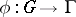satisfying the condition. Ifacts trivially on, then crossed homomorphisms are just ordinary homomorphisms. Crossed homomorphisms are also called-cocycles ofwith values in(see Non-Abelian cohomology). Every elementdefines a crossed homomorphism(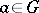), called a principal crossed homomorphism, or cocycle cohomologous to. A mappingis a crossed homomorphism if and only if the mappingofinto the holomorph of(cf. Holomorph of a group) given by, where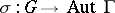is the homomorphism defining theaction on, is a homomorphism. For example, ifis a linear representation ofin a vector space, then any crossed homomorphism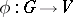defines a representationofby affine transformations of. The set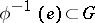is called the kernel of the crossed homomorphism; it is always a subgroup of.